## How to Calculate and Solve for Infinite Acting Period | The Calculator Encyclopedia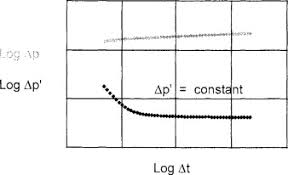The image represents the infinite acting period.

To compute the infinite acting period, six essential parameters are needed and these parameters are permeability (k), porosity (φ), well drainage area (A), viscosity (μ), Dimensionless Time to End of Infinite Acting Period ((tDA)eia) and total compressibility (CT).

The formula for calculating the infinite acting period:

teia = [φ μ CT A / 0.000263K] (tDA)eia

Where;

teia = Infinite Acting Period
K = Permeability
φ = Porosity
A = Well Drainage Area
μ = Viscosity
(tDA)eia = Dimensionless Time to End of Infinite Acting Period
CT = Total Compressibility

Let’s solve an example;
Given that the permeability is 21, porosity is 15, well drainage area is 32, viscosity is 26, dimensionless time to end of infinite acting period is 44 and total compressibility is 34.
Find the infinite acting period?

This implies that;

K = Permeability = 21
φ = Porosity = 15
A = Well Drainage Area = 32
μ = Viscosity = 26
(tDA)eia = Dimensionless Time to End of Infinite Acting Period = 44
CT = Total Compressibility = 34

teia = [φ μ CT A / 0.000263K] (tDA)eia
teia = [15 x 26 x 34 x 32 / 0.000263 x 21] 44
teia = [424320 / 0.005523] 44
teia = [76827810.972] 44
teia = 3380423682.78

Therefore, the infinite acting period is 3380423682.78 s.

## How to Calculate and Solve for Dimensionless Time in Well Testing | The Calculator Encyclopedia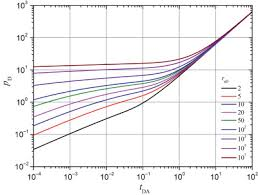The image above represents dimensionless time.

To compute for the dimensionless time, six essential parameters are needed and these parameters are permeability (k), porosity (φ), viscosity (μ), well radius (rw), minimum transient time (t) and total compressibility (CT).

The formula for calculating dimensionless time:

tD = 0.000263kt / φμCTr

Where;

tD = Dimensionless Time
k = Permeability
φ = Porosity
μ = Viscosity
t = Minimum Transient Time
CT = Total Compressibility

Let’s solve an example
Find the dimensionless time when the permeability is 13, porosity is 11.5, viscosity is 10.5, well radius is 24, minimum transient time is 17 with a total compressibility of 28.

This implies that;

k = Permeability = 13
φ = Porosity = 11.5
μ = Viscosity = 10.5
rw = Well Radius = 24
t = Minimum Transient Time = 17
CT = Total Compressibility = 28

tD = 0.000263kt / φμCTr
tD = 0.000263 x 13 x 1711.5 x 10.5 x 28 x 24²
tD = 0.000263 x 13 x 1711.5 x 10.5 x 28 x 576
tD = 0.000263 x 13 x 171947456
tD = 0.0581231947456
tD = 2.9845e-8

Therefore, the dimensionless time is 2.98

Nickzom Calculator – The Calculator Encyclopedia is capable of calculating the dimensionless time.

To get the answer and workings of the dimensionless time using the Nickzom Calculator – The Calculator Encyclopedia. First, you need to obtain the app.

You can get this app via any of these means:

To get access to the professional version via web, you need to register and subscribe for NGN 1,500 per annum to have utter access to all functionalities.
You can also try the demo version via https://www.nickzom.org/calculator

Apple (Paid) – https://itunes.apple.com/us/app/nickzom-calculator/id1331162702?mt=8
Once, you have obtained the calculator encyclopedia app, proceed to the Calculator Map, then click on Petroleum under EngineeringNow, Click on Well Testing under Petroleum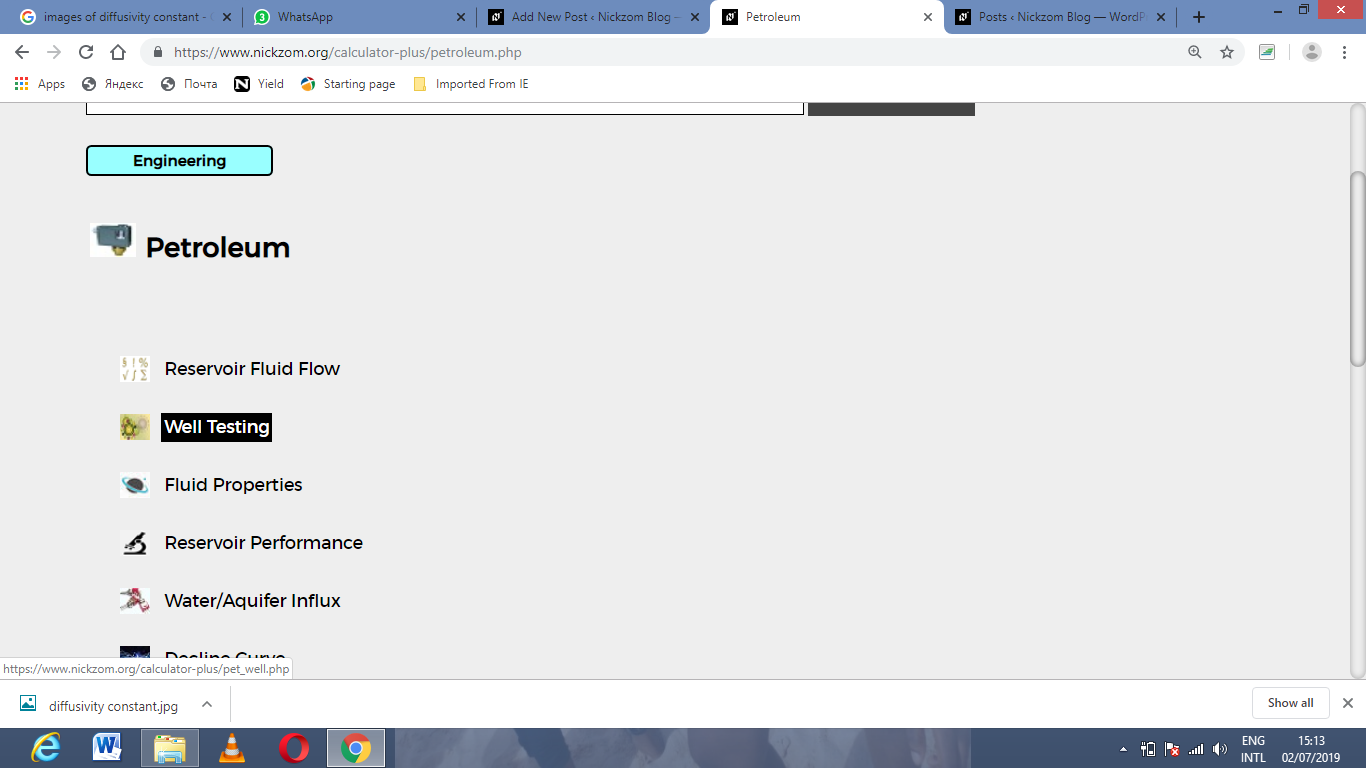Now, Click on Dimensionless Time under Well TestingThe screenshot below displays the page or activity to enter your value, to get the answer for the dimensionless time according to the respective parameter which are the permeability (k), porosity (φ), viscosity (μ), well radius (rw), minimum transient time (t) and total compressibility (CT).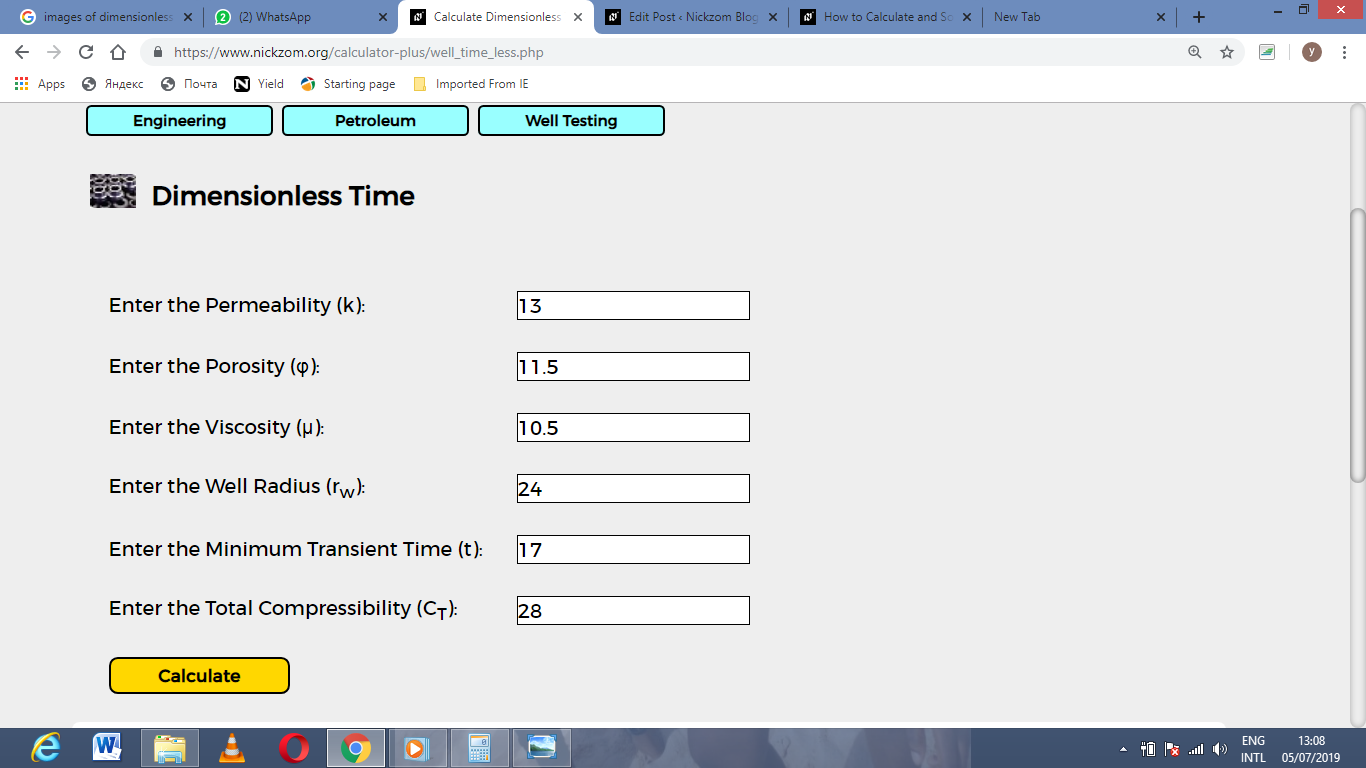Now, enter the value appropriately and accordingly for the parameter as required by the permeability (k) is 13, porosity (φ) is 11.5, viscosity (μ) is 10.5, well radius (rw) is 24, minimum transient time (t) is 17 and total compressibility (CT) is 28.Finally, Click on Calculate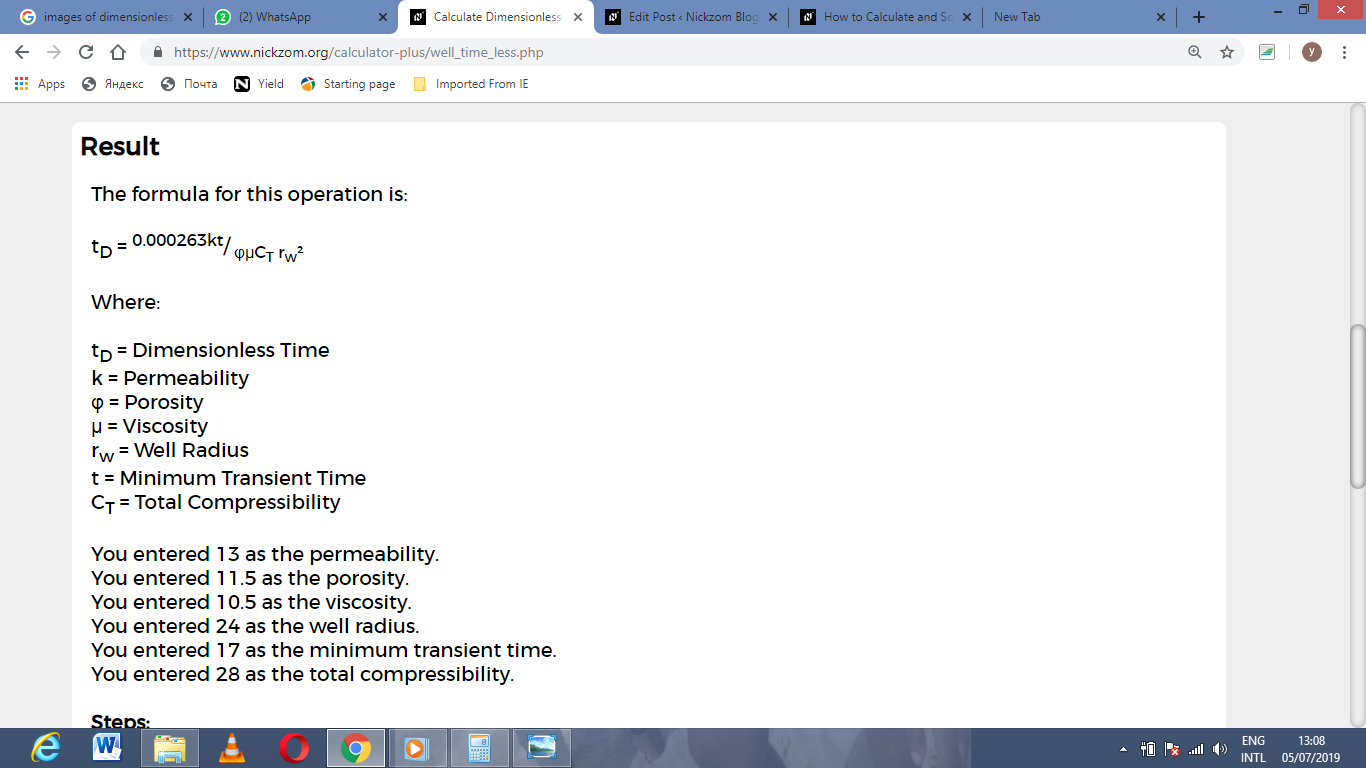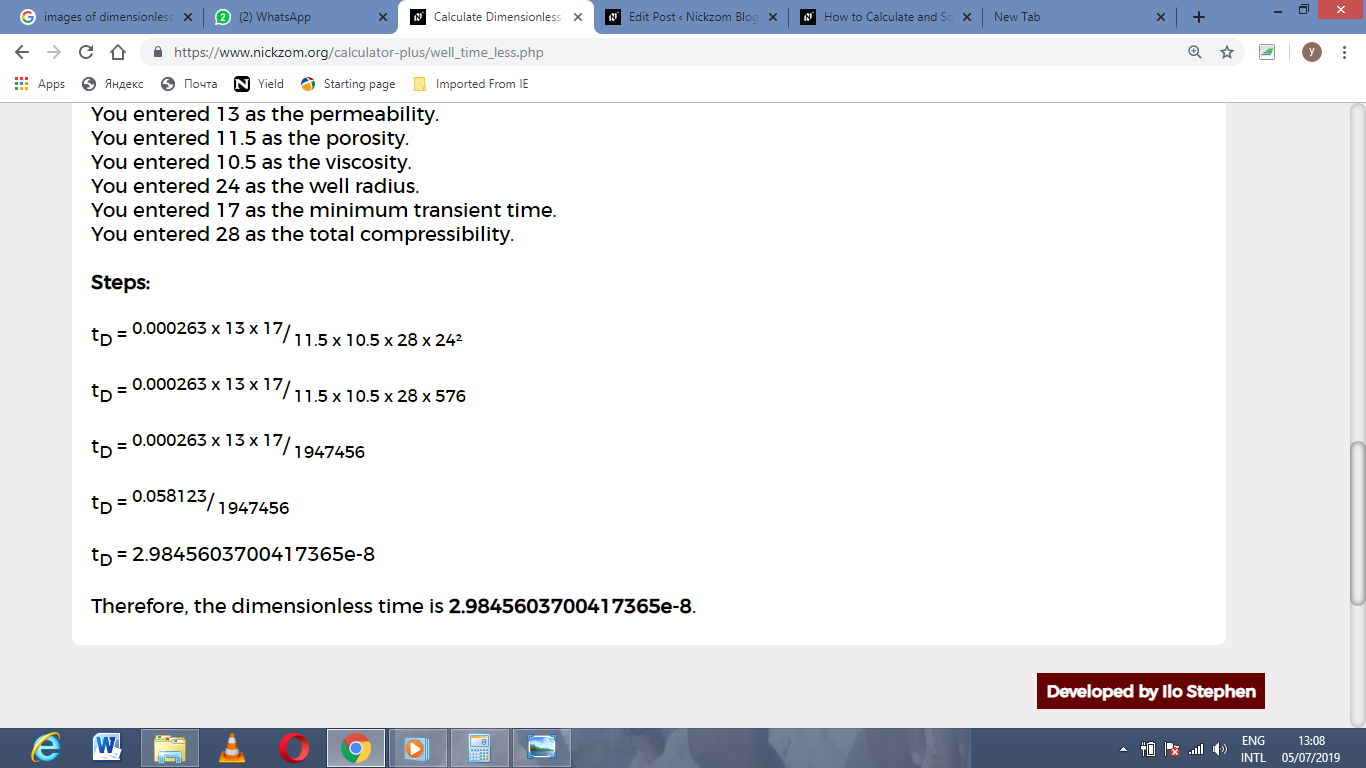As you can see from the screenshot above, Nickzom Calculator– The Calculator Encyclopedia solves for the dimensionless time and presents the formula, workings and steps too.

## How to Calculate and Solve for Minimum Transient Time in Well Testing | The Calculator Encyclopedia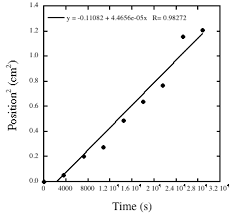The image above represents minimum transient time.

To compute for the minimum transient time, five essential parameters are needed and these parameters are permeability (k), porosity (φ), reservoir radius (r), viscosity (μ) and total compressibility (CT).

The formula for calculating the minimum transient time:

t = 9.48 x 104 φ CT r² / k

Where;

t = Minimum Transient Time
k = Permeability
φ = Porosity
μ = Viscosity
CT = Total Compressibility

Let’s solve an example;
Given that the permeability is 11, porosity is 14, reservoir radius is 7, viscosity is 13 and total compressibility is 22.
Find the minimum transient time?

This implies that;

k = Permeability = 11
φ = Porosity = 14
r = Reservoir Radius = 7
μ = Viscosity = 13
CT = Total Compressibility = 22

t = 9.48 x 104 φ CT r² / k
t = 9.48 x 104 x 14 x 22 x 7² / 11
t = 9.48 x 104 x 14 x 22 x 49 / 11
t = 1430721600 / 11
t = 130065600

Therefore, the minimum transient time is 13006500.

## How to Calculate and Solve for Diffusivity Constant | Well Testing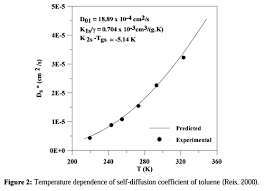The image above represents diffusivity constant.

To compute for the diffusivity constant, four essential parameters are needed and these parameters are pemerability (k), porosity (φ), viscosity (μ) and total compressibility (CT).

The formula for calculating diffusivity constant:

η = 0.000263k / φμCT

Where;

η = Diffusivity Constant
k = Permeability
φ = Porosity
μ = Viscosity
CT = Total Compressibility

Let’s solve an example;
Find the diffusivity constant where the permeability is 12, porosity is 9, viscosity is 7 and total compressibility is 11.

This implies that;

k = Permeability = 12
φ = Porosity = 9
μ = Viscosity = 7
CT = Total Compressibility = 11

η = 0.000263k /  φμCT
η = 0.000263 x 12 / 9 x 7 x 11
η = 0.003156 / 693
η = 0.000004554112554112554

Therefore, the diffusivity constant is 0.000004554112554112554.

Calculating the Permeability when the Diffusivity Constant, Porosity, Viscosity and Total Compressibility is Given.

k = n x φμCT / 0.000263

Where;

k = Permeability
η = Diffusivity Constant
φ = Porosity
μ = Viscosity
CT = Total Compressibility

Let’s solve an example;
Find the permeability where the diffusivity constant is 24, porosity is 10, viscosity is 5 and total compressibility is 13.

This implies that;

η = Diffusivity Constant = 24
φ = Porosity = 10
μ = Viscosity = 5
CT = Total Compressibility = 13

k = n x φμCT / 0.000263
k = 24 x 10 x 5 x 13 / 0.000263
k = 15600 / 0.000263
k = 59315589.3536

Therefore, the permeability is 59315589.3536.

Calculating the Porosity when the Diffusivity Constant, Permeability, Viscosity and Total Compressibility is Given.

φ = 0.000263k / nμCT

Where;

φ = Porosity
k = Permeability
η = Diffusivity Constant
μ = Viscosity
CT = Total Compressibility

Let’s solve an example;
Find the porosity where the diffusivity constant is 28, permeability is 8, viscosity is 12 and total compressibility is 18.

This implies that;

k = Permeability = 8
η = Diffusivity Constant = 28
μ = Viscosity = 12
CT = Total Compressibility = 18

φ = 0.000263k / nμCT
φ = 0.000263 x 8 / 28 x 12 x 18
φ = 0.002104 / 6048
φ = 3.478e-7

Therefore, the porosity is 3.47e-7.

## How to Calculate and Solve for Gas Pore Volume of a Reservoir Fluid Flow | The Calculator Encyclopedia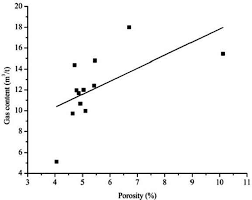The image above represents the gas pore volume.

To compute for the gas pore volume, three essential parameters are needed and these parameters are Initial Gas FVF (Bgi), Initial Water Saturation (Swi) and Initial Gas in Place (G).

The formula for calculating the gas pore volume:

(PV)g = GBgi / 1 – Swi

Where;

(PV)g = Gas Pore Volume
Bgi = Initial Gas FVF
Swi = Initial Water Saturation
G = Initial Gas in Place

Let’s solve an example;
Find the gas pore volume when the initial gas FVF is 12, initial water saturation is 9 and initial gas in place is 13.

This implies that;

Bgi = Initial Gas FVF = 12
Swi = Initial Water Saturation = 9
G = Initial Gas in Place = 13

(PV)g = GBgi / 1 – Swi
(PV)g = 13 x 12 / 1 – 9
(PV)g = 156 / -8
(PV)g =  -19.5

Therefore, the gas pore volume is -19.5

Calculating the Initial Gas FVF when the Gas Pore Volume, Initial Water Saturation and Initial Gas in Place is Given.

Bgi = (PV)g (1 – Swi) / G

Where;

Bgi = Initial Gas FVF
(PV)g = Gas Pore Volume
Swi = Initial Water Saturation
G = Initial Gas in Place

Let’s solve an example;
Find the initial gas FVF when the gas pore volume is 19, initial water saturation is 11 and initial gas in place is 16.

This implies that;

(PV)g = Gas Pore Volume = 19
Swi = Initial Water Saturation = 11
G = Initial Gas in Place = 16

Bgi = (PV)g (1 – Swi) / G
Bgi = 19 (1 – 11) / 16
Bgi = 19 (-10) / 16
Bgi = -190 / 16
Bgi = -11.875

Therefore, the initial gas FVF is -11.875.

Calculating the Initial Water Saturation when the Gas Pore Volume, Initial Gas FVF and Initial Gas in Place is Given.

Swi = 1 – GBgi / (PV)g

Where;

Swi = Initial Water Saturation
G = Initial Gas in Place
(PV)g = Gas Pore Volume
Bgi = Initial Gas FVF

Let’s solve an example;
Find the initial water saturation when the initial gas FVF is 24, initial gas in place is 6 and gas pore volume is 46.

This implies that;

G = Initial Gas in Place = 6
(PV)g = Gas Pore Volume = 46
Bgi = Initial Gas FVF = 24

Swi = 1 – GBgi / (PV)g
Swi = 1 – 6 x 24 / 46
Swi = 1 – 144 / 46
Swi = 1 – 3.13
Swi = -2.13

Therefore, the initial water saturation is -2.13.

## How to Calculate and Solve for Total Formation Volume Factor | The Calculator Encyclopedia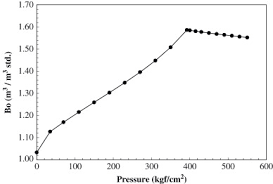The image above represents the total formation volume factor (FVF).

To compute for the total FVF, four essential parameters are needed and these parameters are oil FVF (Bo), gas FVF (Bg), initial gas solubility (Rsi) and current gas solubility (Rs).

The formula for calculating the total FVF:

BT = Bo + (Rsi – Rs)Bg

Where;

BT = Total FVF
Bo = Oil FVF
Bg = Gas FVF
Rsi = Initial Gas Solubility
Rs = Current Gas Solubility

Let’s solve an example;
Find the total FVF when the oil FVF is 14, gas FVF is 15, initial gas solubility is 26 and current gas solubility is 17.

This implies that;

Bo = Oil FVF = 14
Bg = Gas FVF = 15
Rsi = Initial Gas Solubility = 26
Rs = Current Gas Solubility = 17

BT = Bo + (Rsi – Rs)Bg
BT = 14 + (26 – 17)15
BT = 14 + (9)15
BT = 14 + 135
BT = 149

Therefore, the total FVF is 149.

Calculating the Oil FVF when the Total FVF, Gas FVF, Initial Gas Solubility and Current Gas Solubility is Given.

Bo = BT – (Rsi – Rs)Bg

Where;

Bo = Oil FVF
BT = Total FVF
Bg = Gas FVF
Rsi = Initial Gas Solubility
Rs = Current Gas Solubility

Let’s solve an example;
Find the oil FVF when the total FVF is 44, gas FVF is 17, initial gas solubility is 21 and current gas solubility is 19.

This implies that;

BT = Total FVF = 44
Bg = Gas FVF = 17
Rsi = Initial Gas Solubility = 21
Rs = Current Gas Solubility = 19

Bo = BT – (Rsi – Rs)Bg
Bo = 44 – (21 – 19)17
Bo = 44 – (2)17
Bo = 44 – 34
Bo = 10

Therefore, the oil FVF is 10.

Calculating the Gas FVF when the Total FVF, Oil FVF, Initial Gas Solubility and Current Gas Solubility is Given.

Bg = BT – Bo / Rsi – Rs

Where;

Bg = Gas FVF
Bo = Oil FVF
BT = Total FVF
Rsi = Initial Gas Solubility
Rs = Current Gas Solubility

Let’s solve an example;
Find the gas FVF when the total FVF is 48, oil FVF is 16, initial gas solubility is 20 and current gas solubility is 12.

This implies that;

Bo = Oil FVF = 16
BT = Total FVF = 48
Rsi = Initial Gas Solubility = 20
Rs = Current Gas Solubility = 12

Bg = BT – Bo / Rsi – Rs
Bg = 48 – 16 / 20 – 12
Bg = 32 / 8
Bg = 4

Therefore, the gas FVF is 4.

## How to Calculate and Solve for Total Oil Pore Volume of Reservoir Fluid Flow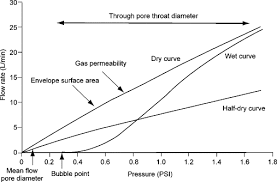The image above represents the total oil pore volume.

To compute for the total oil pore volume, four essential parameters are needed and these parameters are initial oil FVF (Boi), initial water saturation (Swi), oil in place (N) and gas cap ratio (m).

The formula for calculating the total oil pore volume:

(PV)o = NBoi(1 + m) / 1 + Swi

Where;

(PV)o = Total Oil Pore Volume
Boi = Initial Oil FVF
Swi = Initial Water Saturation
N = Oil in Place
m = Gas Cap Ratio

Let’s solve an example;
Find the total oil pore volume when the initial oil FVF is 9, the initial water saturation is 11, the oil in place is 21 and the gas cap ratio is 18.

This implies that;

Boi = Initial Oil FVF = 9
Swi = Initial Water Saturation = 11
N = Oil in Place = 21
m = Gas Cap Ratio = 18

(PV)o = NBoi(1 + m) / 1 + Swi
(PV)o = 21 x 9(1 + 18) / 1 + 11
(PV)o = 3591 / -10
(PV)o = -359.1

Therefore, the total oil pore volume is -359.1

## How to Calculate and Solve for Oil Saturation of Reservoir Fluid Flow | The Calculator Encyclopedia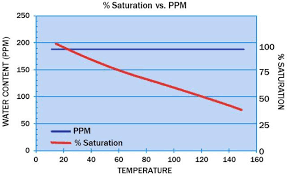The image above represents the oil saturation.

To compute for the oil saturation, five essential parameters are needed and these parameters are cumulative oil production (Np), oil in place (N), initial water saturation (Swi), initial oil FVF (Boi) and oil FVF (Bo).

The formula for calculating oil saturation:

S = [1 – Swi] [1 – Np / N] [Bo / Boi]

Where;

S = Oil Saturation
Np = Cumulative Oil Production
N = Oil in Place
Swi = Initial Water Saturation
Boi = Initial Oil FVF
Bo = Oil FVF

Let’s solve an example;
Given that the cumulative oil production is 22, oil in place is 8, initial water saturation is 16, initial oil FVF is 12 and the oil FVF is 7.
Find the oil saturation?

This implies that;

Np = Cumulative Oil Production = 22
N = Oil in Place = 8
Swi = Initial Water Saturation = 16
Boi = Initial Oil FVF = 12
Bo = Oil FVF = 7

S = [1 – Swi] [1 – Np / N] [Bo / Boi]
S = [1 – 16] [1 – 22/8] [7/12]
S = [1 – 16] [1 – 22/8] [0.583]
S = [1 – 16] [1 – 2.75] [0.583]
S = [1 – 16] [-1.75] [0.583]
S = [-15] [-1.75] [0.583]
S = 15.31

Therefore, the oil saturation is 15.31.

## How to Calculate and Solve for Cumulative GOR of Reservoir Fluid Flow | The Calculator Encyclopedia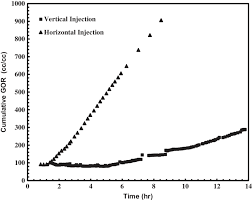The image above represents the cumulative GOR.

To compute for the cumulative GOR, two essential parameters are needed and these parameters are cumulative gas production (Gp) and cumulative oil production (Np).

The formula for calculating the cumulative GOR:

Rp = Gp / Np

Where;

Rp = Cumulative GOR
Gp = Cumulative Gas Production
Np = Cumulative Oil Production

Let’s solve an example;
Find the cumulative GOR with a cumulative gas production of 32 and a cumulative oil production of 44.

This implies that;

Gp = Cumulative Gas Production = 32
Np = Cumulative Oil Production = 44

Rp = Gp / Np
Rp = 32 / 44
Rp = 0.72

Therefore, the cumulative GOR is 0.72.

Calculating the Cumulative Gas Production when the Cumulative GOR and the Cumulative Oil Production is Given

Gp = Rp x Np

Where;

Gp = Cumulative Gas Production
Rp = Cumulative GOR
Np = Cumulative Oil Production

Let’s solve an example;
Find the cumulative gas production when the cumulative GOR is 56 and the cumulative oil production is 19.

This implies that;

Rp = Cumulative GOR = 56
Np = Cumulative Oil Production = 19

Gp = Rp x Np
Gp = 56 x 19
Gp = 1064

Therefore, the cumulative gas production is 1064.

## How to Calculate and Solve for Gas Recovery Factor (Production) | Reservoir Fluid Flow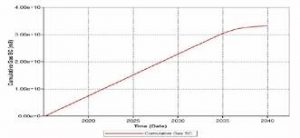The image above represents a gas recovery factor (G).

To compute for a gas recovery factor (production), two essential parameters are needed and these parameters are cumulative gas production (GP) and initial gas in place (G).

The formula for calculating the gas recovery factor (G):

RFG = Gp / G

Where:

RFG = Gas Recovery Factor (G)
GP = Cumulative Gas Production
G = Initial Gas in Place

Let’s solve an example;
Find the gas recovery factor (G) when the cumulative gas production is 13 and the initial gas in place is 17.

This implies that;

GP = Cumulative Gas Production = 13
G = Initial Gas in Place = 17

RFG = Gp / G
RFG = 13 / 17
RFG = 0.764

Therefore, the gas recovery factor (G) is 0.764.

Calculating the Cumulative Gas Production when the Gas Recovery Factor (G) and the Initial Gas in Place is Given.

GP = RFG x G

Where;

GP = Cumulative Gas Production
RFG = Gas Recovery Factor (G)
G = Initial Gas in Place

Let’s solve an example;
Find the cumulative gas production when the gas recovery factor (G) is 24 and the initial gas in place is 6.

This implies that;

RFG = Gas Recovery Factor (G) = 24
G = Initial Gas in Place = 6

GP = RFG x G
GP = 24 x 6
GP = 144

Therefore, the cumulative gas production is 144.